# Classical Geometry Challenges

•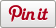• Sharebar
•## 2D Shapes

 No Shape name Description Solutions 1 Regular Triangle A triangle that has three equal sides and angles. 2 Right Angle Triangle A triangle that has a right angle. 3 Isosceles Triangle A triangle that has two equal sides. 4 Scalene Triangle A triangle that has three sides in different lengths. 5 Square A 2D shape that has exactly four 90-degree internal angles and four equal sides. 6 Rectangle Exactly 2 pairs of parallel sides, exactly 4 pairs of adjacent angles are congruent. 7 Kite At least 2 pairs of adjacent sides are congruent, at least 1 pair of opposite angles is congruent. 8 Rhombus Exactly 2 pairs of parallel sides, exactly 4 pairs of adjacent sides are congruent, exactly 2 pairs of opposite angles are congruent. 9 Parallelogram Exactly 2 pairs of parallel sides. 10 Trapezium At least 1 pair of parallel sides. 11 Pentagon 5 sided polygon. 12 Hexagon 6 sided polygon. 13 Heptagon 7 sided polygon. 14 Octagon 8 sided polygon. 15 Nonagon 9 sided polygon. 16 Decagon 10 sided polygon. 17 n-gon Need to provide a LOGO procedure that can create n-gon with specified n.

## 3D Shapes

 No Shape name Description Solutions 1 Cube /node/121 2 Cylinder 3 Cone 4 Sphere 5 Torus 6 Triangular Prism 7 Rectangular Prism 8 Pentagonal Prism 9 n-gonal Prism 10 Anti-Prism 11 Triangular Pyramid 12 Rectangular Pyramid 13 Pentagonal Pyramid 14 n-gonal Pyramid

## Platonic Solids

 No Shape Description Solutions 1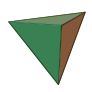Tetrahedron /node/124 2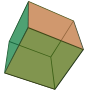Hexahedron /node/121 3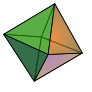Octahedron /node/125 4Dodecahedron /node/127 5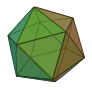Icosahedron /node/94

## Archimedean solid

https://en.wikipedia.org/wiki/Archimedean_solid